# Program to check Buzz Number In Java

In this tutorial you will learn about the Program to check Buzz Number In Java and its application with practical example.

In this tutorial, we will learn to create a Java Program to check Buzz Numbers in Java using Java programming.

## Prerequisites

Before starting with this tutorial we assume that you are best aware of the following Java programming topics:

• Java Operators.
• Basic Input and Output function in Java.
• Class and Object in Java.
• Basic Java programming.
• If-else statements in Java.
• For loop in Java.

## What is Buzz Number?

A Buzz number is a Special number that either ends with 7 or divisible by 7.

Example: Buzz Number

• The number 14 is Buzz number because it is divisible by 7.
• The number 17 is Buzz number because it ends with 7.
• And  number 13 is not a Buzz number because it is neither end with 7 nor divisible by 7.

## Program to check Buzz Number In Java

In this program we will find given number is a Buzz number or not in using java programming. We would first declared and initialized the required variables. Next, we would prompt user to input the a number.Later we  find number is Buzz number or not let’s have a look at the code.

## Output

Buzz Number.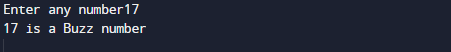Not a Buzz Number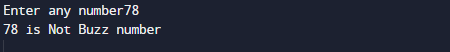In the above program, we have first declared and initialized a set variables required in the program.

• number= it will hold entered number.

After declaring variables in the next statement user will be prompted to enter a value and  which will be assigned to variable ‘number’.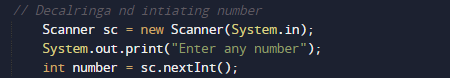Steps to find Buzz number.

1. first take a number from user at run time.
2. then we going to find the last digit within the number and it is divisible by 7 or not. If at least one of condition satisfied then the given number is a “Buzz Number”.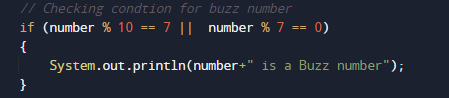Else print “number is not a buzz number”.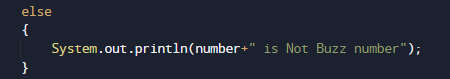In other words, a number is called  Buzz if it ends with 7 or is divisible by 7.

In this tutorial we have learn about the Program to check Buzz Number In Java and its application with practical example. I hope you will like this tutorial.